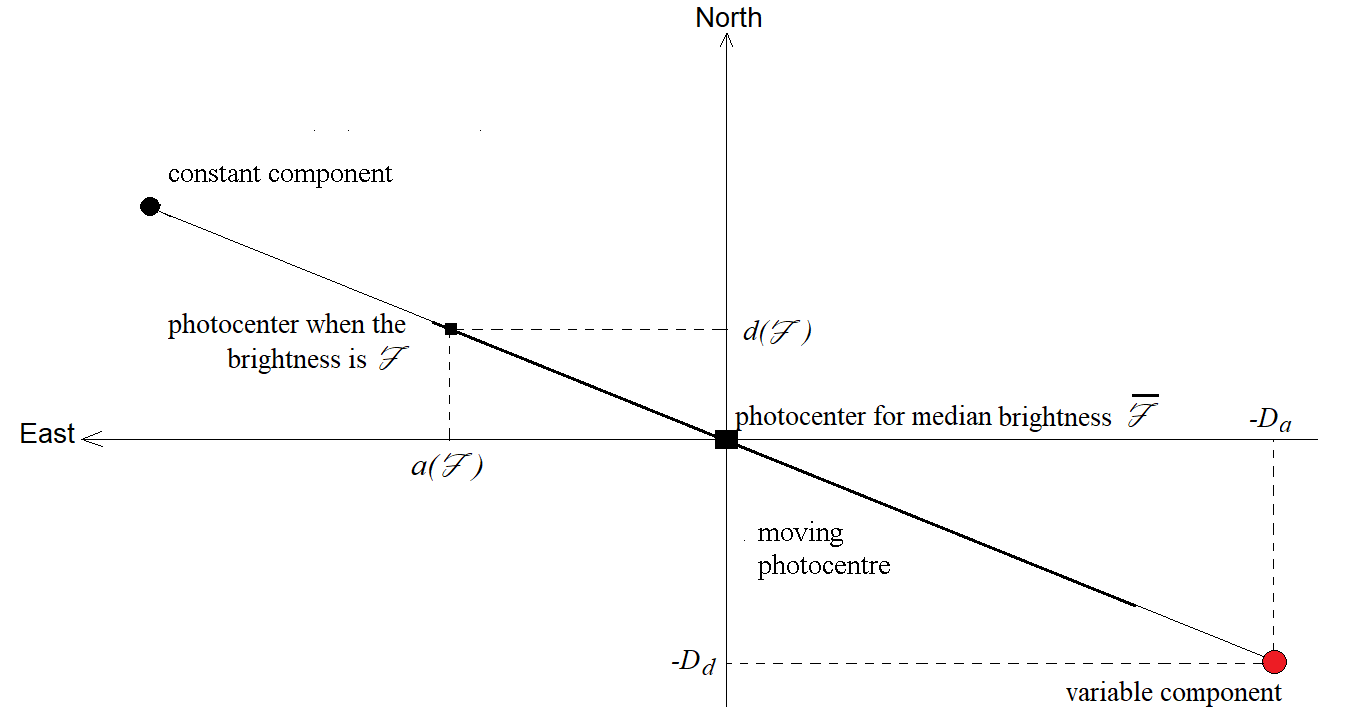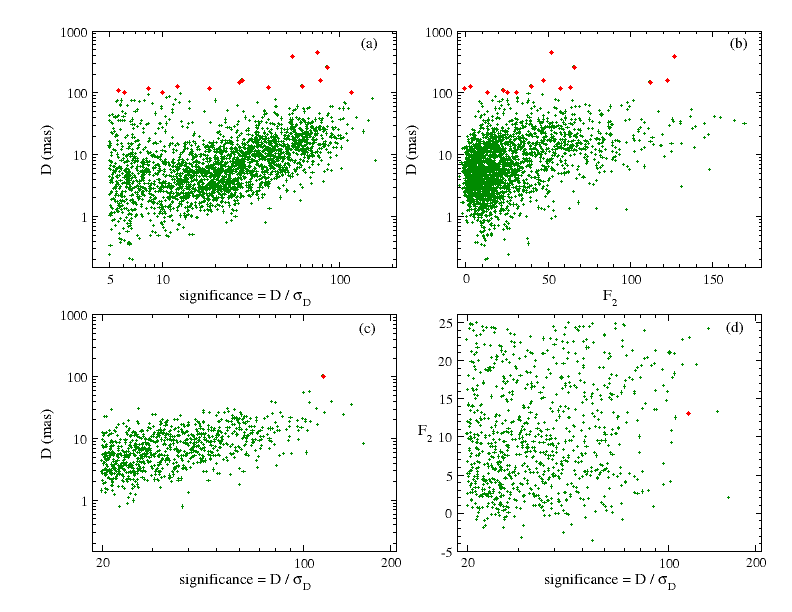# 7.2.6 The variability-induced movers (VIM)

## Selection of the variable stars

By definition, VIMs are photometrically variable, unresolved double stars detected through the displacement of their photocentre. To avoid having to sort out unusable solutions, the calculation has been restricted to photometrically variable stars. These were detected on the basis of a $\chi^{2}$ test, using $\mathrm{varGaussG}$ defined by:

 $\mathrm{varGaussG}=\sqrt{2\chi^{2}}-\sqrt{2(n_{\mathrm{mes}}-n_{\mathrm{par}})% -1}$ (7.25)

where $\chi^{2}$ is calculated from the G-band fluxes, their mean value and their uncertainties, $n_{\mathrm{mes}}$ is the number of measurements and $n_{\mathrm{par}}$ is the number of estimated parameters, i.e. 1 (the mean flux value). It is known that, for $n_{\mathrm{mes}}-n_{\mathrm{par}}$ beyond 30, $\mathrm{varGaussG}$ follows the Gaussian distribution ${\cal N}(0,1)$ (Johnson 1994).

We started by applying the VIM models on stars of $\mathrm{varGaussG}>3$, but it appeared that the false solution rate decreased significantly when applying much larger limits. For this reason, the VIM models were finally tried on stars of $\mathrm{varGaussG}>1\,000$.

## The VIM with Fixed component (VIMF) modelFigure 7.8: A VIM system with Fixed components in the local reference frame. The photocentre is moving between the two components, and the solution refers to its position when the photometric flux is the median ℱ¯. Additional parameters are Da and Dd, the coordinates of the photocentre for flux ℱ¯ measured from the variable component. The separation between the components cannot be derived, but the position angle of the constant star, θ is derived from Da and Dd.

A Variability-Induced Mover (VIM) is a double star with a photometrically variable component. As the pair is not resolved, the position of the photocentre moves between the components in relation to the total brightness in the $G$ photometric band (see Figure 7.8). For consistency with Wielen (1996) and with (ESA 1997, volume 1, Section 2.3.5), the photocentre is located from the variable component, and call $(D_{a},D_{d})$ the coordinates of the photocentre when the total photometric flux of the system is equal to its median value, $\bar{\cal F}$. Therefore, the coordinates of the variable component from the position of the photocentre when the brightness is median are $(-D_{a},-D_{d})$. Wielen (1996) has shown that, when the flux of photocentre is ${\cal F}$, the photocentre coordinates $(a({\cal F}),d({\cal F}))$ are given by the equation:

 $\begin{array}[]{rl}a({\cal F})=&\left(\frac{\bar{\cal F}}{\cal F}-1\right)D_{a% }\\ &\\ d({\cal F})=&\left(\frac{\bar{\cal F}}{\cal F}-1\right)D_{d}\end{array}$ (7.26)

When the components are fixed with respect to each other, $D_{a}$ and $D_{d}$ are constant and are unknowns of the VIM with Fixed component (VIMF) model. The along-scan abscissa of the photocentre of a VIMF system is given by the following equation:

 $w=w_{\mathrm{ss}}+\;\frac{\partial w}{\partial a}\left(\frac{\bar{\cal F}}{% \cal F}-1\right)D_{a}+\frac{\partial w}{\partial d}\left(\frac{\bar{\cal F}}{% \cal F}-1\right)D_{d}$ (7.27)

where $w_{\mathrm{s}s}$ is the same as in Section 7.2.4, so there are 7 unknowns in total. As the single star model is linear, the calculation of the solution amounts to solving a system of linear equations. However, unlike previous models, the uncertainty in $w$ is no longer the only uncertainty in the astrometric measurement, but the uncertainty in the flux, $\sigma_{\cal F}$, must also be taken into account. By differentiating Equation 7.27, one may see that an error in ${\cal F}$ induces the following error in $w$:

 $\sigma_{\mathrm{mod}}=\sigma_{\cal F}\;\frac{\bar{F}}{F^{2}}\;\left|\left(% \frac{\partial w}{\partial a}D_{a}+\frac{\partial w}{\partial d}D_{d}\right)\right|$ (7.28)

The uncertainty that is assigned to $w$ is the quadratic sum of $\sigma_{\mathrm{mod}}$ and of the uncertainty of the astrometric measurement. As the calculation of $\sigma_{\mathrm{mod}}$ depends on $D_{a}$ and $D_{d}$, and thus on the VIMF solution, the calculation of the latter is done in two iterations.

## Selection of the VIMF solutionsFigure 7.9: The VIMF solutions. Panels (a) and (b) refer to the 2508 solutions with significance sVIMF>5 obtained from the processing. Panels (c) and (d) refer to the 870 solutions eventually retained, with sVIMF>20 and F⁢2<25. The red dots are the solutions with D>100 mas.

The significance of the VIMF solutions is defined as the ratio between the norm of the $(D_{a},D_{d})$ vector divided by its uncertainty. Adapting Equation 7.6 to the VIMF terms, one obtains:

 $s_{\mathrm{VIMF}}=\frac{1}{\sigma_{D_{a}}\sigma_{D_{d}}}\sqrt{\frac{D_{a}^{2}% \sigma_{D_{d}}^{2}+D_{d}^{2}\sigma_{D_{a}}^{2}-2D_{a}D_{d}\rho_{D_{a}D_{d}}% \sigma_{D_{a}}\sigma_{D_{d}}}{1-\rho^{2}_{D_{a}D_{d}}}}$ (7.29)

Since the VIMF model was the last model to be tried, the acceptance criterions were those of the alternative solution, i.e. $s_{\mathrm{VIMF}}>5$ and $F2<1\,000$, provided that no other model has produced an alternative solution with a smaller $F2$. However, the acceptance condition $\varpi/\sigma_{\varpi}>30$ was added to guarantee the astrometric quality of the solutions.

To estimate whether a solution is acceptable, there are no other criteria than the modulus of $D$, i.e. $D=\sqrt{D_{a}^{2}+D_{d}^{2}}$. Since the separation between unresolved components is limited to about 100 mas, it is unlikely that one will find VIMF systems with $D>100$ mas. This value is rather conservative, since it assumes a separation of the order of 100 mas, but also a variable component of negligible luminosity when it is at its minimum, and much brighter than the photometrically constant component when it is at its maximum. The distributions of the solutions in the diagrams $s_{\mathrm{VIMF}}$ vs. $D$ and $F2$ vs. $D$ are shown in the upper panels of Figure 7.9. The significance vs. $D$ diagram clearly shows an ascending sequence, with significance ranging from 10 to 100, and which seems to correspond to correct solutions, with the largest $D$ distances being logically the most significant. The $F2$ vs. $D$ diagram shows a clump for $F2<30$. For consistency with previous models, the selection conditions: $F2<25$ and $s_{\mathrm{V}IMF}>20$ was finally applied. The lower part of Figure 7.9 shows the properties of the 870 solutions selected. Only 3 solutions with $D>40$ mas remain, the largest value being $D=101$ mas.

## Other VIM models

The VIMF model is only the first of five different VIM models. In order of increasing complexity (and decreasing orbital period), we have tried:

• The VIM with linear motion model (VIML), which is an extension of the VIMF model by adding the time derivatives of $D_{a}$ and $D_{d}$.

• Two VIM with acceleration models (VIMA). Like the acceleration models seen in Section 7.2.4, the first of these models includes a constant acceleration, while the second adds the derivative of the acceleration with respect to time.

• The orbital VIM model (VIMO).

All these models have been included in the preliminary versions of the processing, and have been tested on simulations. It was found that these models involved far fewer objects than the VIMF model: starting from ideal simulated measurements, VIML solutions were already 88 times fewer than VIMF, and more complex solutions were even more scarce. The use of real data made things even worse: from the VIML model it is possible to calculate the minimum masses of the binaries, and contrary to the simulations, the result was devastating: not only are the masses very large (usually more than 100 solar masses), but it was impossible to select credible mass binaries on the basis of quality criteria, such as model significance or parallax significance. Therefore, the solutions from the VIML models and beyond were all rejected.